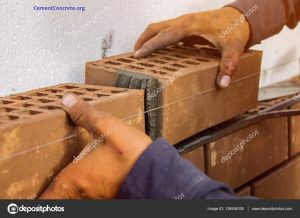October 1, 2023

## Brick Calculator for walls, Columns, foundations| Brick estimator

• Home
• Brick Calculator for walls, Columns, foundations| Brick estimator

### Brick Masonry Calculation

Meter
Cm
Meter
Cm
Cm

Size of Brick

cm
Cm
Cm

450

#### Volume of Construction

0.9 m3
SN. Material Quantity
1 Bricks 450
2 Cement 2.13 Bags
3 Sand 0.34 Ton

### Bricks masonry calculation

##### Step 1 :

$$Volume\;of\;Brick\;Masonry = \;Length(m) \times Depth(m) \times Wall\;Thickness(m)$$

$$Volume\;of\;Brick\;Masonry = \;3 \times 3 \times 0.1$$

$$Volume\;of\;Brick\;Masonry = 0.9\;{m^3}$$

$$Brick\;Size = 19(cm) \times 9(cm) \times 9(cm)$$

$$Brick\;Size = 19(100) \times 9(100) \times 9(100)$$

$$Brick\;Size = 0.19(m) \times 0.09(m) \times 0.09(m)$$

$$Size\;of\;Brick\;with\;Mortor = (0.19 + 0.01) \times (0.09 + 0.01) \times (0.09 + 0.01)$$

$$Size\;of\;Brick\;with\;Mortor = 0.2(m) \times 0.1(m) \times 0.1(m)$$

$$No\;of\;Bricks = {{Volume\;of\;Brick\;Mansonary} \over {Volume\;of\;one\;Brick}}$$

$$No\;of\;Bricks = {{0.9\;{m^3}} \over {0.2\times 0.1\times 0.1}}$$

$$No\;of\;Bricks = 450\;Bricks$$

$$Actual\;Volume\;of\;Bricks\;Mortor = No\;of\;Bricks \times Volume of Brick Without Mortor$$

$$Actual\;Volume\;of\;Bricks\;Mortor = 450 \times 0.19 \times 0.09 \times 0.09$$

$$Actual\;Volume\;of\;Bricks\;Mortor = 450 \times (0.0015)$$

$$Actual\;Volume\;of\;Bricks\;Mortor = 0.6926\;{m^3}$$

$$Quantity\;of\;Mortor = Volume\;of\;Brick\;Mansonary - Actual\;Volume\;of\;Bricks\;without\;mortar$$

$$Quantity\;of\;Mortor = 0.9 - 0.6926$$

$$Quantity\;of\;Mortor = 0.2075\;{m^3}$$

Add 15% more for wastage, Non - uniform thickness of mortar joins

$$Quantity\;of\;Mortor = 0.2075 + \left( {0.2075 \times {{15} \over {100}}} \right)$$

--

$$Quantity\;of\;Mortor = 0.2386$$

Add 25% more for Dry Volume

$$Quantity\;of\;Mortor = 0.2386 + \left( {0.2386 \times {{25} \over {100}}} \right)$$

$$Quantity\;of\;Mortor = 0.2982\;{m^3}$$

##### Amount of Cement
$${{Cement} \over {Sum\;of\;Ratio}} \times Quantity\;of\;Mortar$$ $$Cement = {{1} \over {4}} \times 0.2982$$ $$Cement = 0.0746\;{m^3}$$
1 Bag of Cement = 0.035 m3
$$No\;of\;Cement\;Bags = {{0.0746} \over {0.035}}$$ $$No\;of\;Cement\;Bags = 2.13 Bags$$ $$Cement\;in\;kg = 106.5 kg$$
##### Amount of Sand Required
$${{Sand} \over {Sum\;of\;Ratio}} \times Quantity\;of\;Mortar$$ $$Sand = {{3} \over {4}} \times 0.3$$ $$Sand = 0.4$$
By Considering dry loose bulk density of sand 1500 kg/m3
$$Sand = 0.22 \times 1500$$ $$Sand = 335.49 kg$$

### Brick Calculator for walls, Columns, foundations| Brick estimator

Brick calculator is a calculation and conversion tool where you can calculate the number of bricks required for a given brick masonry wall, column,  foundation, or brick pavement area. This brick calculation tool will also calculate the amount of sand and cement required for a given mortar in brick masonry.

After calculating the quantity of brick masonry, we can easily estimate the cost of brick masonry with the rates provided in your locality.Please, read below carefully how the brick calculator works and you can easily estimate the number of bricks and how much mortar you have to manage for your project.

### Other consideration facilities

If you are involved in the field of construction industries and need to calculate how many bags of cement are required and the quantity of sand, and aggregate required for concrete, you’ll be better off using our concrete calculator.

### Process (Step) of Brick mortar calculation:

The followings are the steps used for the brick calculator tool:

Came on the screen of our concrete calculation tool above.

#1. First, select the unit. In metric system(m, cm) or United states Standards Feet system(Feet, inch).

#2. Put the value of length and height (depth) of your masonry unit

#3. Select the wall thickness.

#4. Select the mortar ratio (cement, sand, ratio) as 1:3, 1:4,1:5, 1:6, 1:7, 1:8 etc. fr your work needed.

[Recommendation:  for half brick masonry 1:4, for brick masonry 1:6 to 1:5]

#5. Select the size of the brick according to your standard norms. [in US, 7.5 inch x 3.5 inch x 3.5 inch  and in India 190 mm x 90 mm x  90 mm].

#6. Then press the calculate button.

#7. There will be results showing no. of bricks, cement bags and sand required in ton or m3.

Hence, this way we can easily calculate the brick for your project.

## Brick masonry calculation formula

The volume of brick masonry = Length x Depth x thickness of the wall

Brick volume with mortar = Brick length x Brick width x Brick hight

No of Bricks = (Volume of brick masonry)/ Volume of one Brick

## The standard size of bricks

In the United States brick size is 7.5 inches x 3.5 inch x 3.5 inch with mortar. In India 190 mm x 90 mm x  90 mm without mortar. The mortar thickness is ½ inch in the US, and 10 mm in the metric system.

With the mortar thickness, the size of brick becomes (8inch x 4 inch x 4 inch) in the US and (200mm x 100 mm x100 mm ) in the metric system.

### For example for Brick calculation in a masonry wall

In the metric system:

Let us consider, a masonry wall of 6m in length, 4m height, and 230 mm in thickness.

• Volume of brick in masonry = 6x4x0.23 = 5.52 m3
• Volume of brick with mortar = 0.2×0.1×0.1 = 0.002 m3
• Number of bricks = (Volume of brick)/(Volume of one brick) = 2760 bricks.

Hence, you can say the of brick per cubic meter is 500.

By this method, you can calculate no. of brick inn column, foundation, footing, pavement, etc.

### United states standard system in yards

Let s consider, a wall of 20 feet length, 10 feet hight and 5 inch thicknesss.

Now, volume of brick wall = 20x 10x 5/12 = 83.33 cubic feet = 3.086 cubic yards.

Volume of brick with mortar = 8x4x4= 128 cubic inch = 0,074074 cubic feet

Now, number of brick required = masonary volume/ volume of  1 brick

= 83.33/0.074074 = 1125 no. of bricks.

Hence, the no. of brick required per cubic feet is 13.50, similarly, no. of brick per cubic yard is 365 number.

Civil engineering Calculator Tools: